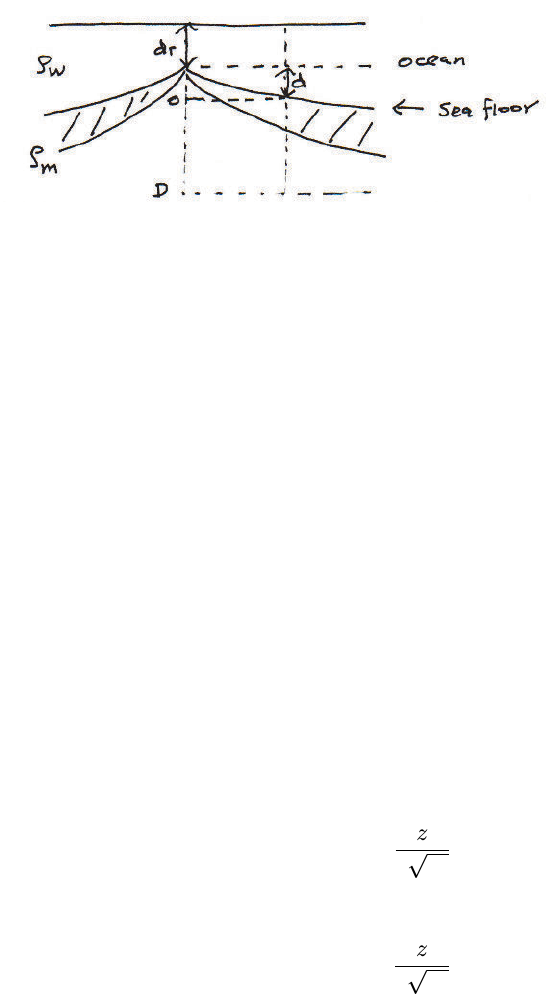Study Guides (400,000)
US (230,000)
UCSB (3,000)
EARTH (10)
Final

# EARTH 135 Lecture Notes - Lecture 13: Center For Retrospective Digitization, Thermal Expansion, Multiple IntegralExam

Department
Earth Science
Course Code
EARTH 135
Professor
Tanimoto
Study Guide
Final

This preview shows page 1. to view the full 4 pages of the document.Change in ocean depth for cooling halfspace model
Test of the Cooling Halfspace Model (Section 4.23 in TS)
In this lecture, we are going to see a test of the cooling oceanic lithosphere
model. This model can explain an increasing ocean depth with age of the
ocean ﬂoor.
Let us compare the pressure under the ridge axis and the pressure under
the ocean ﬂoor at age t. We write the depth to the ridge axis as drand ocean
depth at age tas dr+d(t). We take the depth coordinate zdownward positive
and its origin (z= 0) as shown in the ﬁgure.
Pressure in the deep interior, say at z=D, should be equal whether it is
under the age zero or under the age t. Then we can write
ρwgdr+ρmg(d+D) = ρwg(dr+d) + ZD
0
ρgdz (1)
where ρwis density of sea water and ρmis density of deep mantle. Density ρ
in the integrand is a function of depth zbecause as the plate cools with age,
its density increases due to thermal contraction.
Density ρat temperature Tcan be written
ρ=ρm+ρmα(TmT) (2)
where αis the thermal expansion coeﬃcient. We can write Tas
T(z, t) = Ts+ (TmTs)erf(z
2κt) (3)
Then TmTin (2) can be written
TmT= (TmTs)(1 erf(z
2κt)) (4)
1